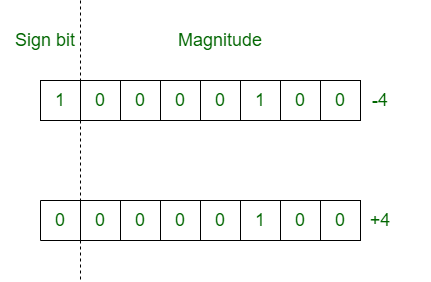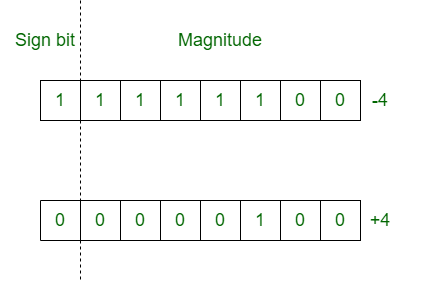Open In App

# Difference between Signed magnitude and 2’s complement

1. Signed Magnitude Method :
In the signed magnitude method number is divided into two parts: Sign bit and magnitude. Sign bit is 1 for negative number and 0 for positive number. Magnitude of number is represented with the binary form of the number.
Example: Lets take 8 bits register.2. 2’s Complement Method :
In 2’s complement method, positive numbers are represented in the same way as they are represented in sign magnitude method. But if the number is negative, first represent the number with positive sign and then take 2’s complement of that number.
Example: Lets take 8 bits register.Difference between Signed Magnitude and 2’s Complement Method :

SIGNED MAGNITUDE METHOD2’s COMPLEMENT METHOD
It is a method to denote fixed point signed numbers.It is also used to denote fixed point signed numbers.
Number is divided into two parts.Number is considered as a whole.
Sign bit is considered explicitly.Sign bit is not considered explicitly.
Additional hardware is required for resultant sign of arithmetic.No additional hardware is required in 2’s complement method.